Next: MINIMUM K-SUPPLIER Up: Miscellaneous Previous: MINIMUM K-CLUSTERING   Index

### MINIMUM K-CLUSTERING SUM

• INSTANCE: Finite set X, a distance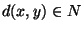for each pair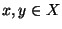. The distances must satisfy the triangle inequality.

• SOLUTION: A partition of X into disjoint subsets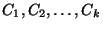.

• MEASURE: The sum of all distances between elements in the same subset, i.e.,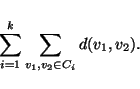• Good News: Approximable within 2 .

• Comment: If the triangle inequality is not satisfied the problem is called MINIMUM EDGE DELETION K-PARTITION. Approximable within 1.7 for k=2 . The maximization variation in which the subsets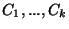must be of equal size c is approximable within 2(c-1)/c or (c+1)/c, depending on whether c is even or odd  Moreover, if d does not satisfy the triangle inequality then the bounds are c-1 and c, respectively ; the cases c=3 and c=4 are approximable within 2 . Finally, if required sizes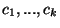of the subsets are given and the triangle inequality is satisfied then the maximization problem is approximable within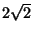.Next: MINIMUM K-SUPPLIER Up: Miscellaneous Previous: MINIMUM K-CLUSTERING   Index
Viggo Kann
2000-03-20